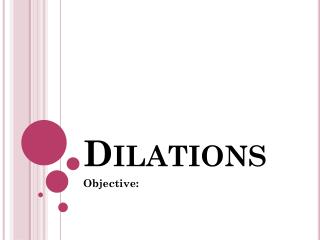Download PresentationDilations

Dilations - PowerPoint PPT Presentation

Dilations. Objective: . Dilation. A Dilation can enlarge or reduce a figure by a scale factor . . What is a Dilation ? A dilation is a transformation that changes the size but not the shape of the figure. We use “  ” to identify new points. . Scale Factor. What is a Scale Factor?I am the owner, or an agent authorized to act on behalf of the owner, of the copyrighted work described.
Download PresentationDilations

Download Policy: Content on the Website is provided to you AS IS for your information and personal use and may not be sold / licensed / shared on other websites without getting consent from its author.While downloading, if for some reason you are not able to download a presentation, the publisher may have deleted the file from their server.

- - - - - - - - - - - - - - - - - - - - - - - - - - E N D - - - - - - - - - - - - - - - - - - - - - - - - - -
Presentation Transcript
1. Dilations Objective:

2. Dilation A Dilation can enlarge or reduce a figure by a scale factor. • What is a Dilation? A dilation is a transformation that changes the size but not the shape of the figure. We use “  ” to identify new points.

3. Scale Factor • What is a Scale Factor? A scale factor is scale that enlarges or reduces similar figures, can be expressed as a decimal, a fraction, or percent. Example: A 10% increase is a scale factor of 1.1 and a 10% decrease is a scale factor of 0.9.

4. Helpful Hints!! A scale factor between 0 and 1 reduces a figure. A scale factor greater than 1 enlarges it. Scale Factor = 1/2 Scale Factor = 2

5. Try This!!! Tell whether each transformation is a dilation. The transformation is a dilation The transformation is not a dilation

6. A' A A' B' B B' C' C C' A B C Try This!!! Tell whether each transformation is a dilation. The transformation is a dilation The transformation is not a dilation. The figure is changed.

7. Center of Dilation • What is the center of Dilation? A center of dilation is a fixed point of every dilation, found by drawing a line that connects each of the pairs of corresponding vertices.

8. Try This!!! Dilate the figure by a scale factor of 0.4 with P as the center of dilation. Multiply each side by 0.4 P and P’ are the same point

9. Try This!!! Dilate the figure by a scale factor of 1.5. What are the vertices of the image? Multiply the coordinates by 1.5 to find the vertices of the image The vertices of the image are

10. Try This!!! Dilate the figure by a scale factor of . What are the vertices of the image? Multiply the coordinates by to find the vertices of the image The vertices of the image are

11. Example 1:

12. Example 2:

13. Example 3: Dilate the figure by a scale factor of 2. What are the vertices of the image? 10 8 6 C 4 2 A B 10 8 4 6 2 0

14. Example 4: Dilate the figure by a scale factor of 0.5. What are the vertices of the image? 10 C 8 6 B A 4 2 10 8 4 6 2 0